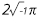# People

 Kyoji Saito PositionVisiting Senior Scientist (from 2017/04/01 ) show past_positionsOther AffiliationResearch Institute for Mathematical Sciences, Kyoto University Research FieldMathematics Mathematics E-Mail  URLhttp://www.kurims.kyoto-u.ac.jp/ja/list/saito-k.htmlLast Update 2022/11/21

To understand the arc length 2π of a unit circle is one of the most ancient mathematical subject. Since the unit circle C is given by the quadratic equation x2+y2=1, using the complex variable z=x+iy, one hasThis integral is called a period integral, since the inverse function of the indefinite integral ∫dz/z is the exponential function with periodThis period integral can be described by the Lie algebra of type A1. Period integrals over complex curves defined by cubic or quartic equations are called elliptic integrals, whose inversion functions are the elliptic functions. These elliptic integrals can be described by the Lie algebras of type A2, B2 and G2 of rank 2. In thisway, several interesting mathematical structures are crossing in the study of period integrals. In order to construct a higher dimensional generalization of the elliptic integrals, called the integral of primitive forms, categorically, I introduced certain infinite dimensional Lie algebras associated with infinite root systems. The flat structure (or, Frobenius manifold structure) and the flat coordinates, which appeared as a byproduct of this study, is nowadays one of the standard languages used to describe mirror symmetry. It is an important thema to determined the automorphic forms obtained by inverting the period integral maps.

Back to Member List.